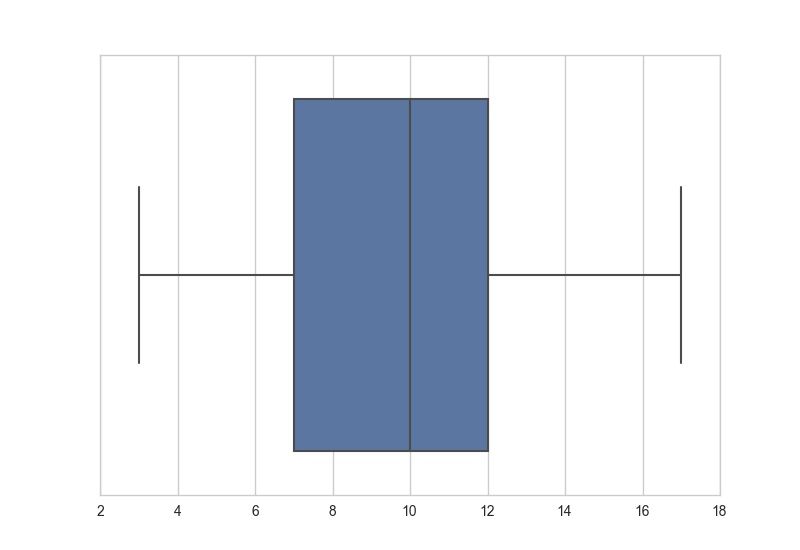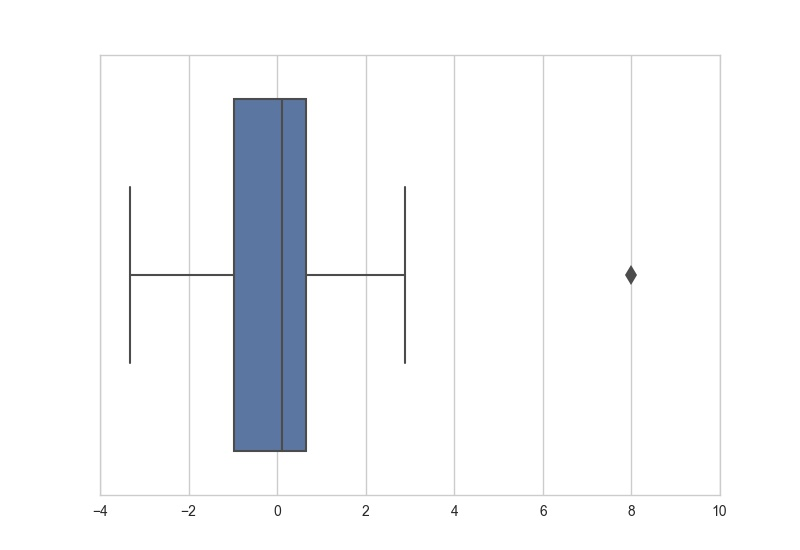Probability

# Box PlotsWhich of the following is closest to the largest value in the data set represented by the box plot above?Consider the box plot above which was generated from a set of 9 values. If the largest possible mean of the numbers (i.e. their arithmetic average) is $m$, which is closest to $9m$?

Hint: think of what values the box plot tells us must be in the data set.The box plot above represents a series of measurements. Which is closest to the largest measurement?What is the mean of the numbers in the data set represented by the box plot above (note: the mean of some numbers is the arithmetic average of them).Let the box plot above represent a very big data set. Which is closest to the percentage of the data points in the set of data that are less than or equal to 7?

×